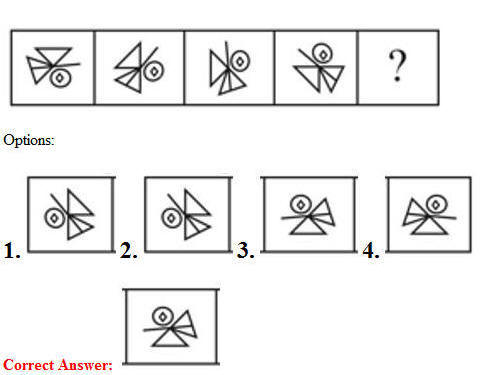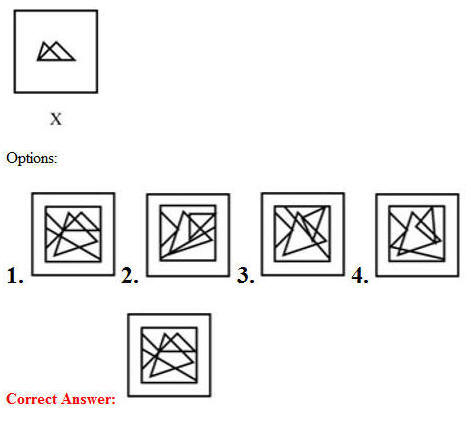# 11 July 2019 – Evening-Shift (Reasoning)

SSC CHSL (Tier – 1) Online Exam Paper – 2018 “held on 11 JULY 2019”

## Evening-Shift (Reasoning)

Q1. Which number will replace the question mark (?) in the following series?
2, 3, 8, 31, ?

Options:

1. 95
2. 154
3. 105
4. 134

Q2. A paper is folded and cut as shown below. How will it appear when unfolded?Q3. Select the option that is related to the third letter-pair in the same way as the second letter-pair is related to the first letter-pair.
FU : UF : : RS : ?

Options:

1. JH
2. GH
3. IH
4. IP

Q4. Arrange the following words as they appear in the English Dictionary.

1. Chairman
2. Challan
3. Chaos
4. Champion
5. Changing

Options:

1. 1, 2, 4, 5, 3
2. 1, 4, 2, 3, 5
3. 1, 3, 4, 2, 5
4. 1, 2, 3, 4, 5

Correct Answer: 1, 2, 4, 5, 3

Q5. Select the Answer Fig. that will come next in the following Problem Fig. series.Q6. Select the option that is related to the third letter-pair in the same way as the second letter-pair is related to the first letter-pair.
GP : TK : : DR ?

Options:

1. WX
2. VU
3. IW
4. WI

Q7. Three of the following four words are alike in a certain way and one is different. Pick the odd word out.

Options:

1. Tomato
2. Onion
3. Potato
4. Egg

Q8. ‘Dice’ is related to ‘Cube’ in the same way as ‘Ball’ is related to ‘__________’.

Ans

1. Cone
2. Cube
3. Sphere
4. Line

Q9. In a code language, THRUST is written as UJUYXZ. How will WOOD be written as in that language?

Options:

1. XORH
2. XQRH
3. XQRI
4. XQSH

Q10. Select the set in which the numbers are related in the same way as are the numbers of the following set.
(35, 48, 83)

Options:

1. (25, 49, 91)
2. (24, 49, 75)
3. (15, 24, 39)
4. (15, 25, 41)

Q11. Three statements are given, followed by three conclusions numbered I, II and III. Assuming the statements to be true, even if they seem to be at variance with commonly known facts, decide which of the conclusions logically follow(s) from the statements.

Statements:
All knifes are axes.
All axes are swords.
All saws are swords.

Conclusions:
I. All swords are knifes.
II. Some swords are axes.
III. Some swords are saws.

Options:

1. Only conclusions II and III follow.
2. All the conclusions follow.
3. Only conclusions I and II follow.
4. Only conclusion I follows.

Correct Answer: Only conclusions II and III follow.

Q12. Shyam is the brother of Riya. Mohini is the mother of Anuj. Riya is the daughter of Ram. Anuj is the brother of Shyam. How is Mohini related to Ram?

Options:

1. Mother
2. Wife
3. Daughter
4. Sister

Q13. Select the correct mirror image of the given figure is placed to the right of the figure.Q14. Three of the following four numbers are alike in a certain way and one is different.
Pick the number that is different from the rest.

Options:

1. 8715
2. 6915
3. 8915
4. 7815

Q15. Select the Venn diagram that best illustrates the relationship between the following classes.
Lawyers, Architects, Human BeingsQ16. Select the number-pair in which the two numbers are related in the same way as are the two numbers of the following number-pair.
20 : 30

Options:

1. 12 : 23
2. 16 : 36
3. 30 : 42
4. 6 : 14

Q17. Which two signs should be interchanged in the following equation to make it correct?
10 + 18 – 20 × 100 ÷ 5 = 27

Options:

1. × and –
2. + and –
3. × and ÷
4. + and ÷

Q18. If it was a Saturday on 10 November 2018, what was the day of the week on 15 August 2017?

Options:

1. Friday
2. Sunday
3. Tuesday
4. Monday

Q19. Three of the following four letter-clusters are alike in a certain way and one is different. Pick the odd one out.

Options:

1. PTX
2. DHL
3. NRV
4. FKM

Q20. How many squares are present in the following figure:1. 21
2. 19
3. 17
4. 23

Q21. Which letter-pair will replace the question mark (?) in the following series?
KH, ? , QN, WT, EB

Options:

1. MK
2. MJ
3. NJ
4. LN

Q22. Select the option in which the given figure is embedded.Q24. Select the set in which the numbers are related in the same way as are the numbers of the following set.
(10, 32, 54)

Options:

1. (25, 47, 77)
2. (35, 57, 79)
3. (15, 49, 37)
4. (16, 37, 56)